###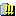Author Topic: I'm a Genius. Are you?  (Read 8617 times)

0 Members and 1 Guest are viewing this topic.

####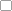lexicon

• Jr. Poster
•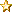• Posts: 3840
• Respect: +196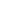##### Re: I'm a Genius. Are you?
« Reply #15 on: September 08, 2016, 03:10:15 PM »
123 + 0?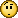Like this post: 0

#### bulbasaur

• Guest##### Re: I'm a Genius. Are you?
« Reply #16 on: September 08, 2016, 03:22:41 PM »
More examples...

2+1=103
9+7=216
8+5=313

Just because you figured out Part 1, that doesn't mean that you actually answered Part 2.  I don't want to tell too much because that would be giving away the answer.

Like this post: 0

#### bulbasaur

• Guest##### Re: I'm a Genius. Are you?
« Reply #17 on: September 08, 2016, 03:25:46 PM »
More examples...

22+10=1232
15+13=228
17+12=529

Like this post: 0

#### bulbasaur

• Guest##### Re: I'm a Genius. Are you?
« Reply #18 on: September 08, 2016, 03:33:07 PM »
The way this problem was originally proposed to me was starting with the question in Part 2 first.  That makes the problem a little more challenging.

Like this post: 0

#### bulbasaur

• Guest##### Re: I'm a Genius. Are you?
« Reply #19 on: September 08, 2016, 04:43:40 PM »
It is not impressive to simply know the answer.  It is more impressive to be able to solve it.  Showing a completed 10,000 piece puzzle is not impressive if you didn't put it together yourself.  The same thing goes for many things in life.  Showing me a great art piece is fine, but that doesn't make you impressive  or a genius.  The person who made the art is impressive and a genius.

Like this post: 0

#### bulbasaur

• Guest##### Re: I'm a Genius. Are you?
« Reply #20 on: September 08, 2016, 04:47:49 PM »
Or, think of it this way....

You have a math book.  The book comes with an answer guide.  Just because you have all the answers, that does not make you good at the math that is in the book.

Like this post: 0

#### bulbasaur

• Guest##### Re: I'm a Genius. Are you?
« Reply #21 on: September 08, 2016, 05:40:51 PM »
I remember those several times where you couldn't think logically.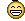Dont you remember when we went to school together and you had to do a math problem on the board?

https://youtu.be/MTghfMCU1rY

Like this post: 0

#### bulbasaur

• Guest##### Re: I'm a Genius. Are you?
« Reply #22 on: September 08, 2016, 10:53:42 PM »
Hint: 12 and 11 is just one possible answer.  There is more than one answer.  You're supposed to find all possible answers.

Like this post: 0

####lexicon

• Jr. Poster
•• Posts: 3840
• Respect: +196##### Re: I'm a Genius. Are you?
« Reply #23 on: September 09, 2016, 08:03:11 AM »
Hint: 12 and 11 is just one possible answer.  There is more than one answer.  You're supposed to find all possible answers.

I figured as much. But all I can think of now is how many points will my RB's get me this Sunday?

I'll revisit this later today.

Like this post: 0

####lexicon

• Jr. Poster
•• Posts: 3840
• Respect: +196##### Re: I'm a Genius. Are you?
« Reply #24 on: September 09, 2016, 11:30:04 AM »
I think I have it.

In X + XX = 123, 123 dictates the value X and XX in only part A or SUBTRACTION. The value of X and XX remain as they are for part B ADDITION.

Since 123 = 3 digits = hundreds. Should 2 digits = tens?

(X*100)+(X*100)=123
1200+1100=123
1200-1100=100 SUBTRACTION
Overall 100+23=123

Examples;

2+1=103
103= 3 digits = hundreds
200-100=100
2+1=3
100+3=103

SO...
5+1=46
46= 2 digits= tens
50-10=40
5+1=6
40+6=46« Last Edit: September 09, 2016, 12:17:50 PM by lexicon »

Like this post: 0

####Believe_N_Me

• Elite Poster
•• Posts: 13518
• Respect: +444##### Re: I'm a Genius. Are you?
« Reply #25 on: September 05, 2022, 04:30:58 AM »
So a friend at work posed this question to us.  Apparently you need a high IQ to figure this out.  I got it pretty quick, and some people were impressed.  That being said, I don't think the problem requires genius intellect.  They probably would have figured it out as well if given enough time.  Anyways, here is the problem...

7+6=113
8+5=313
9+2=711

What's the pattern?  Can you figure it out?

7+6 = 113 (1 = 7-6, 13 = 7+6)
8+5 = 313 (3 = 8-5, 13 = 8+5)
9+2 = 711 (7 = 9-2, 11 = 9+2)

continuing pattern:

5+2 = 37
6+4 = 210
7+6 = 113
8+8 = 016

Like this post: 0